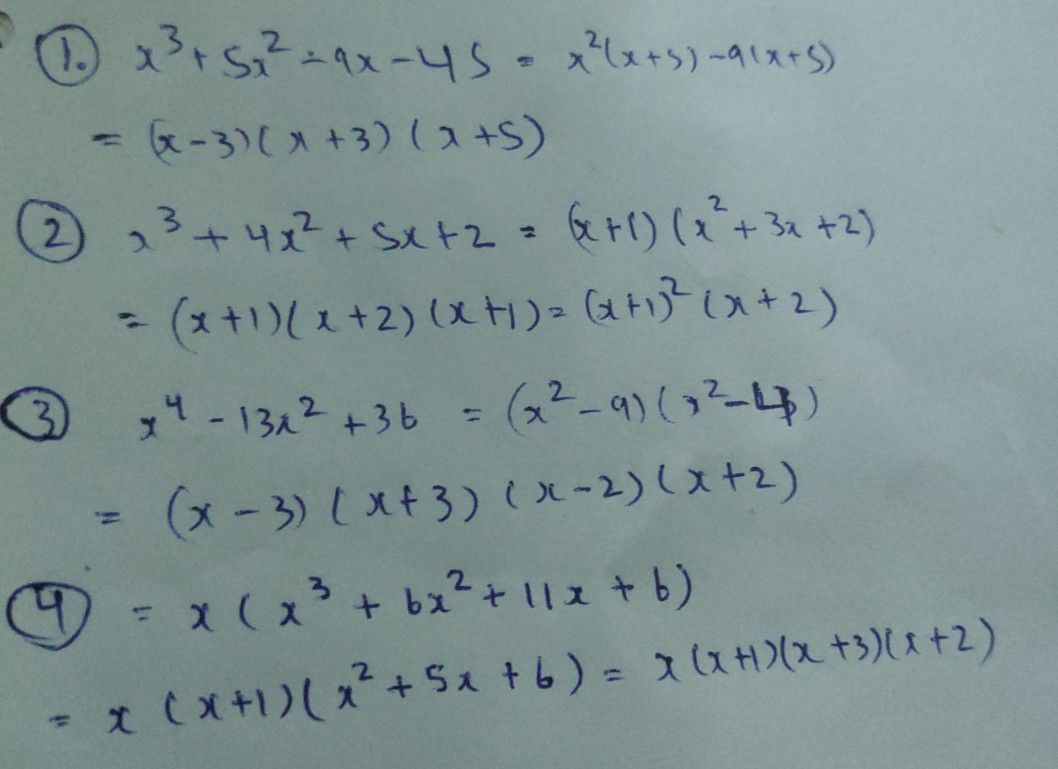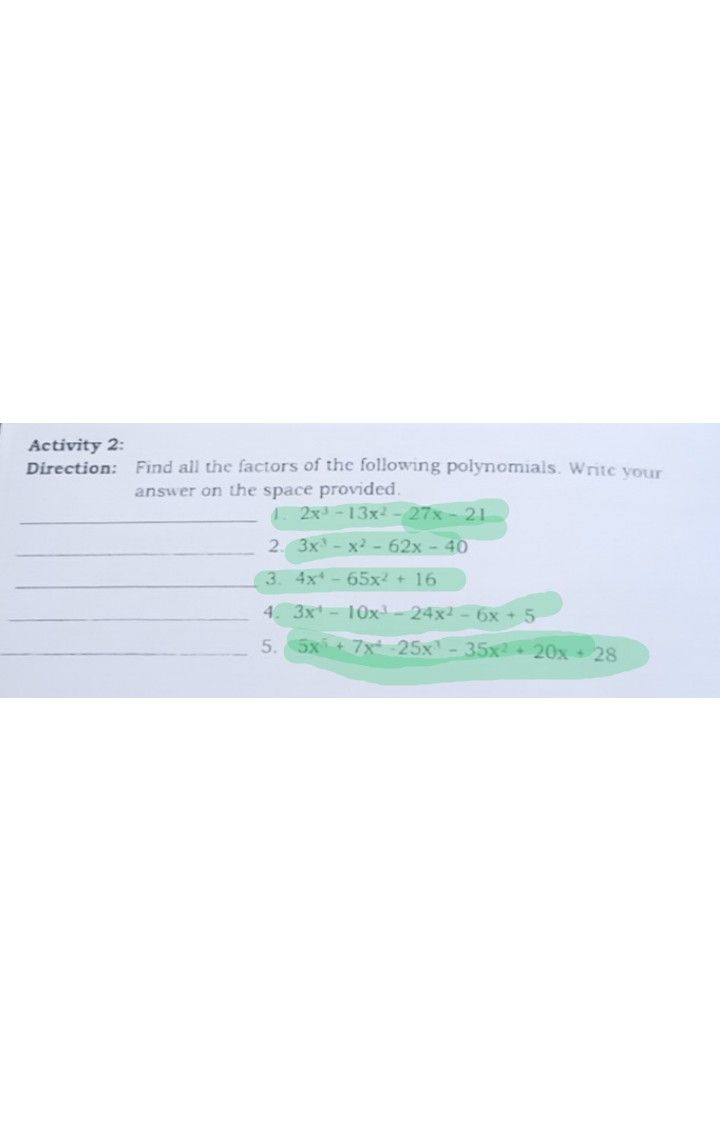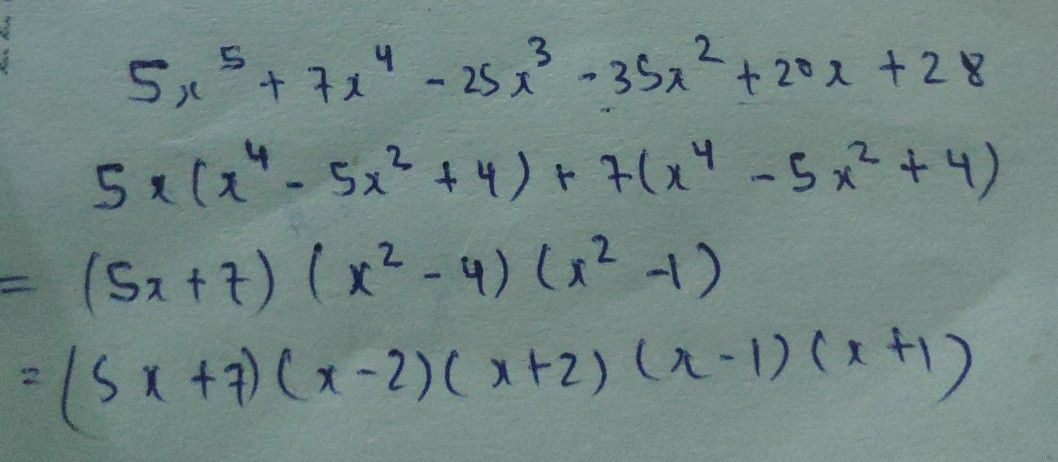Symbol
ProblemActivity $11$ $Di\pi cct1on$ Find all the factors of the following polynomials Write your answer on the space provided. 1. $x3+5x2-9x-45$ - $2$ $x^{3+4x^{2+5x+2}}$ $3$ $x^{4-13x2+36}$ $4$ $x^{4+6x^{3+11x^{2+6x}}}$ $5,$ $x^{3-2x^{4}}-11x^{1}+56x^{2+20x}$ $16$
10th-13th grade
Algebra
Search count: 105
SolutionQanda teacher - PayalStudent
thank you so muchQanda teacher - Payal